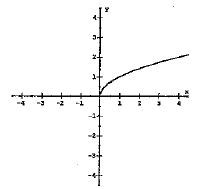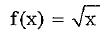# Parent functions - graph and function name. Flashcards

Set Details Share
created 8 years ago by MrWashington
753 views
Matching parent functions.
updated 8 years ago by MrWashington
Subjects:
math
Page to share:
Embed this setcancel
COPY
code changes based on your size selection
Size:
X
1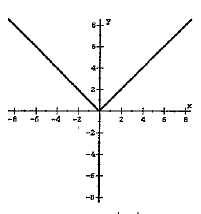f(x) = |x|

2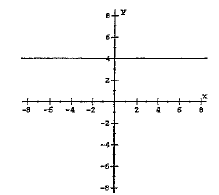f(x) = a

3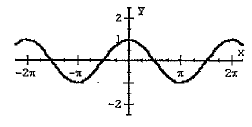f(x) = cosx

4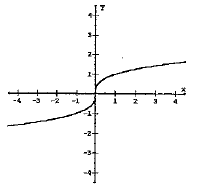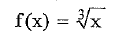5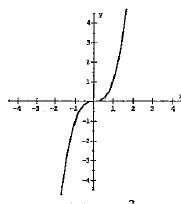f(x) = x3

6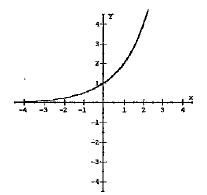f(x) = ax

7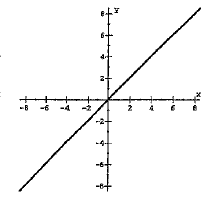f(x) = x

8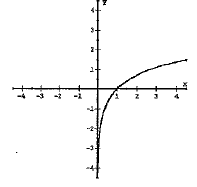f(x) = logax

9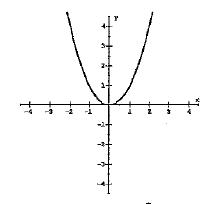f(x) = x2

10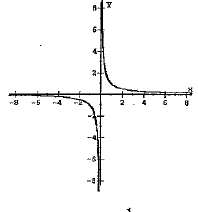f(x) = 1/x

11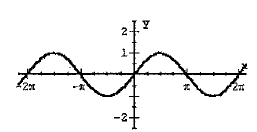f(x) = sinx

12